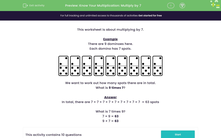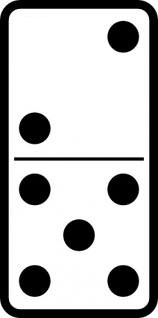# Practise Your Seven Times Table

In this worksheet, students will learn to multiply by 7.Key stage:  KS 2

Curriculum topic:   Number: Multiplication and Division

Curriculum subtopic:   Know Multiplication Tables up to 12 x 12

Difficulty level:#### Worksheet Overview

This activity is about multiplying by 7.

Example

There are 9 dominoes here.

Each domino has 7 spots.We want to work out how many spots there are in total.

What is 9 times 7?

In total, there are 7 + 7 + 7 + 7 + 7 + 7 + 7 + 7 + 7  = 63 spots

What is 7 times 9?

7 × 9 = 63

9 × 7 = 63

That's it!

So long as we know our seven times table, this activity will be easy!### What is EdPlace?

We're your National Curriculum aligned online education content provider helping each child succeed in English, maths and science from year 1 to GCSE. With an EdPlace account you’ll be able to track and measure progress, helping each child achieve their best. We build confidence and attainment by personalising each child’s learning at a level that suits them.

Get started••••